# GMAT Quant: Geometry – Quadrilaterals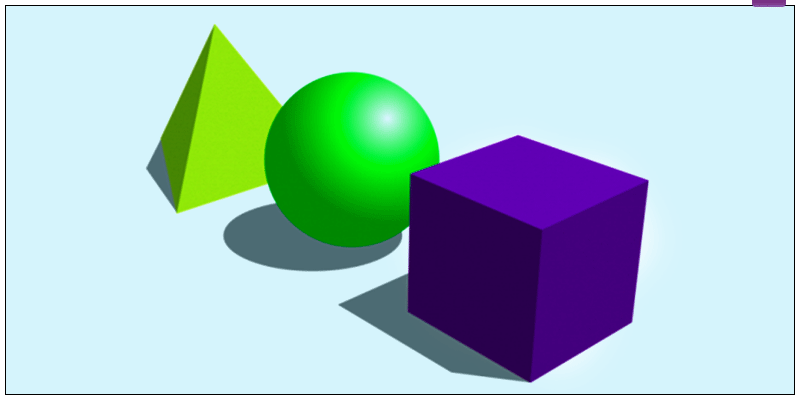In GMAT exam, Geometry questions are the most scoring one. Few basic concepts and formulas can help you solve geometry questions in faction of seconds. Here are few Geometry Sample Questions for GMAT Exam. Let’s learn all about Quadrilaterals.

Any figure that has four sides can be termed as a quadrilateral. There can be many forms of quadrilateral, such as, rhombus, square, rectangle, trapezium, parallelogram etc.

Types of quadrilateral and their properties:

1. Square

• All sides are equal and spread at an angle of 90o. While, its opposite sides are parallel to one another.
• The diagonals of a square are equal and bisect one another at an angle of 902. Rectangle• The opposite sides of a rectangle are parallel and equal.
• All sides are spread at an angle of 90o
• The diagonals are equal in length.
• The diagonals of a rectangle bisect each other at 90o.
• A rectangle is a special type of parallelogram whose all sides are spread at an angle of 90o.

3. Parallelogram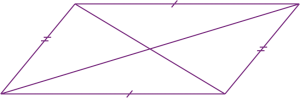• The diagonals of a parallelogram bisect one another.
• Opposite sides of a parallelogram are equal and parallel.
• In a parallelogram, opposite angles are equal.
• The sum of adjacent angle is equal to 180o.

4. Rhombus• All sides of a rhombus are equal.
• Only the opposite angles are equal.
• The sum of adjacent angles is supplementary
• The diagonals bisect one another at complementary angle.

5. Kite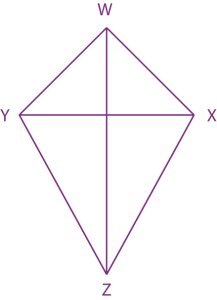• The adjacent sides of a kite are equal in length
• The diagonals bisect one another at complementary angle.
• OX = OY (Where O is the point of intersection)

6. Trapezoid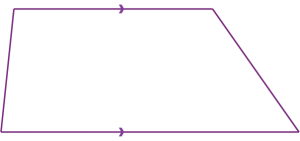• Trapezoid is a four sided figure which has only one pair of parallel sides
• The sum of adjacent sides is equal to 180o.

The most favorite quadrilateral figure of GMAT is parallelogram.

So, what exactly is a parallelogram?

A parallelogram is a four sided figure whose opposite sides are equal and parallel to one another and only the opposite angles are equal.

Let’s solve some questions to see how GMAT plans on confusing its aspirants.

Question: Calculate the area of the parallelogram: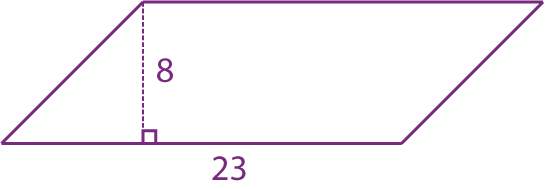Solution: $Area \; of \; parallelogram \; = \; base \times height$

Here, height = 8 cm and base = 23 cm

So, $Area = \; 8 \times 23 = 184 \; cm^{2}$

We’ll be glad to help you in your GMAT preparation journey. You can ask for any assistance related to GMAT and MBA from us by calling us at +918884544444. You can write to us at gmat@byjus.com.